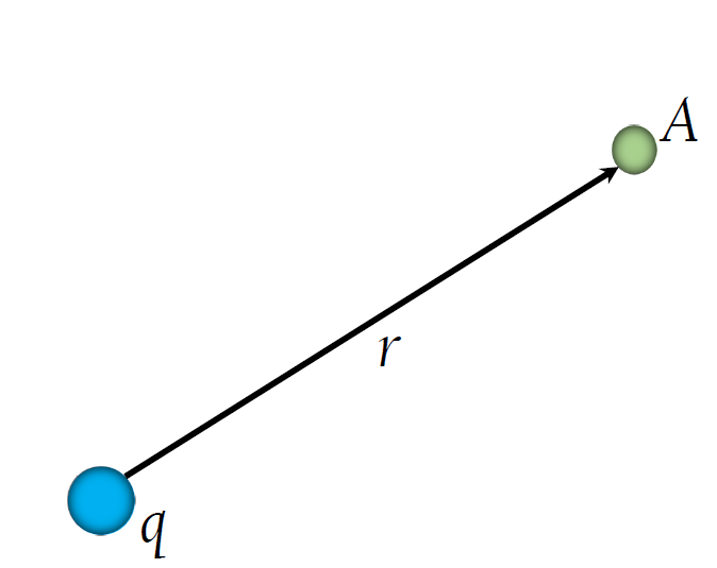# Electric Potential Calculator

Created by Luciano Mino
Last updated: Aug 24, 2022

Our electric potential calculator can obtain the electric potential at any distance from a single point charge or a number of point charges (up to ten).

If you don't know what the electric potential is, don't worry. Within this short article, we will cover:

• Electric potential definition;
• Electric potential formula/equation;
• Electric potential due to a point charge; and
• Electric potential due to a system of point charges.

Let's start!

## What is the electric potential? Electric potential formula

We define the electric potential of a point charge at a point in space as the work required to bring a $1\ \text{C}$ charge from infinity to that location.

Sounds confusing? Let's do some math first so you can start working with the electric potential calculator.

💡 Alternatively, check our electric field calculator to get a proper introduction to this topic.

Assume we had two positive point charges, $q$ and $Q$, separated by a distance of $r$.

If you remember, Coulomb's law describes the force $F$ between these particles:

$F = \frac{k_{e}qQ}{r^{2}}$

where $k_{e}$ is Coulomb's constant.

Now, assume we tried to bring the charges closer by moving $q$ from $r_{1}$ to $r_{2}$ ($r_{1} > r_{2}$). For that, we would need to do work against this repulsive force:

$dW = -\frac{k_{e}qQ}{r^{2}}dr$

by integrating between $r_{1}$ and $r_{2}$ we get:

$W_{r_{1}r_{2}}=k_{e}qQ(\frac{1}{r_{2}}-\frac{1}{r_{1}})$

Lastly, if we replace one of the charges with a unit test charge (a $1\ \text{C}$ positive charge) and set our initial point at infinity, we obtain the electric potential equation for a point charge:

$V(r) =\frac{k_{e}Q}{r}$

This potential depends only on $r$ and $Q$, and its unit is the volt (V).

🔎 Electromagnetism is an interesting topic with some counterintuitive repercussions, such as the force between current-carrying wires. Read more about it in this calculator!

## Electric potential due to a system of point charges

We can extend this definition to a system of multiple point charges easily.

The electric potential of multiple point charges $q_{i}$ at a point in space is simply the vector sum of each individual electric potential:

$V(r) = k_{e} \sum_{i}\frac{q_{i}}{|r-r_{i}|}$

With our electric potential calculator, you can input up to ten point charges and it will output the resulting electric potential at any point. Give it a try!

🙋 Our electric potential calculator is straightforward: input the charge and the distance, and it will automatically output the electric potential at that position.

Luciano Mino
I want to calculate.....
Electric potential
due to a point chargeCharge (q)
C
Distance (r)
μm
Electric potential (V)
x10⁶
V
People also viewed…

### Free space path loss

The free space path loss calculator allows you to find the strength of a radio frequency signal emitted by an antenna at any given distance.

### Magnetic force on current-carrying wire

Find the strength of the electromagnetic force of the magnetic field around a straight wire using this Magnetic Force on a Current-Carrying Wire Calculator.